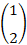-->

# Linear Independence in vector space

Definition.
The vectors v1, v2, ..., v3 in the vector v space are called linearly independent if

c1v1 + c2v2 + ... + cnvn = 0

resulting in all scalars c1, c2, ..., c3 must be equal to 0.

Example 1.are linearly independent, because if

Then,
c1 +   c2 = 0
c1 + 2c2 = 0
And the only solution to this system is c1 = 0, c2 = 0.

Definition.
Vectors v1, v2, ..., vn in vector v space are called linearly dependent if there are scalars c1, c2, ..., cn which are not all zero so
c1v1 + c2v2 + ... + cnvn = 0

Example 2.
Determine what vectors are
v1 = (1, -2, 3)     v2 = (5, 6, -1)   v3 = (3, 2, 1)
linear Independent or linearly dependent?

Solution:
On the vector component segment
c1v1 + c2v2 + c3v3 = 0
to be
c1= (1, -2, 3) + c2(5, 6, -1)   + c3(3, 2, 1) = (0, 0, 0)
or equivalent to
(c1 + 5c2 + 3c3, -2c1 + 6c2 + 2c3, 3c1 – c2 + c3) = (0, 0, 0)
By equalizing the corresponding component will give
c1 + 5c2 + 3c3 = 0
2c1 + 6c2 + 2c3 = 0
3c1 – c2 + c3 = 0
By completing this system it will produce
c1= -1/2t              c2 = -1/2t                    c3 = t
So, this system has non-trivial solutions, so the three vectors are linearly dependent.

Theorem 1.
Suppose x1, x2, ..., xn are n vectors in Rn and for example
x1 = (x1i, x2i, ..., xni)T untuk i = 1, 2, ..., n
if X = (x1, x2, ..., xn) then vectors x1, x2, ..., xn are linearly dependent if and only if X is singular.

Proof:
The equation c1x1 + c2x2 + ... + cnxn = 0 is equivalent to the system of equations

If we say c = (c1, c2, ..., cn)T, then this system can be written as a matrix equation.
Xc = 0
This equation will have a non-trivial solution if and only if X is singular. So x1, x2, ..., xn will depend linearly if and only if X is singular.

Example 3.
Determine whether vectors (4, 2, 3)T,  (2, 3, 1)T and (2, -5. 3)T are linearly dependent or not.

Solution:
Because

Then these vectors are linear dependent.